University Physics Volume 2

# Conceptual Questions

University Physics Volume 2Conceptual Questions

### Conceptual Questions

1.

A stationary coil is in a magnetic field that is changing with time. Does the emf induced in the coil depend on the actual values of the magnetic field?

2.

In Faraday’s experiments, what would be the advantage of using coils with many turns?

3.

A copper ring and a wooden ring of the same dimensions are placed in magnetic fields so that there is the same change in magnetic flux through them. Compare the induced electric fields and currents in the rings.

4.

Discuss the factors determining the induced emf in a closed loop of wire.

5.

(a) Does the induced emf in a circuit depend on the resistance of the circuit? (b) Does the induced current depend on the resistance of the circuit?

6.

How would changing the radius of loop D shown below affect its emf, assuming C and D are much closer together compared to their radii?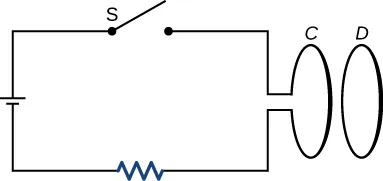7.

Can there be an induced emf in a circuit at an instant when the magnetic flux through the circuit is zero?

8.

Does the induced emf always act to decrease the magnetic flux through a circuit?

9.

How would you position a flat loop of wire in a changing magnetic field so that there is no induced emf in the loop?

10.

The normal to the plane of a single-turn conducting loop is directed at an angle $θθ$ to a spatially uniform magnetic field $B→.B→.$ It has a fixed area and orientation relative to the magnetic field. Show that the emf induced in the loop is given by $ε=(dB/dt)(Acosθ),ε=(dB/dt)(Acosθ),$ where A is the area of the loop.

### 13.2Lenz's Law

11.

The circular conducting loops shown in the accompanying figure are parallel, perpendicular to the plane of the page, and coaxial. (a) When the switch S is closed, what is the direction of the current induced in D? (b) When the switch is opened, what is the direction of the current induced in loop D?12.

The north pole of a magnet is moved toward a copper loop, as shown below. If you are looking at the loop from above the magnet, will you say the induced current is circulating clockwise or counterclockwise?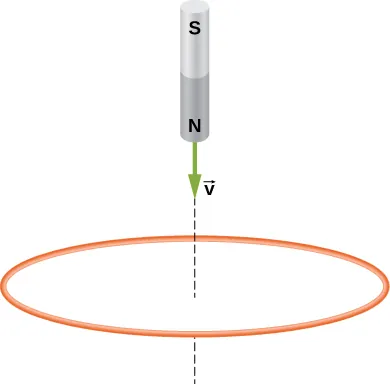13.

The accompanying figure shows a conducting ring at various positions as it moves through a magnetic field. What is the sense of the induced emf for each of those positions?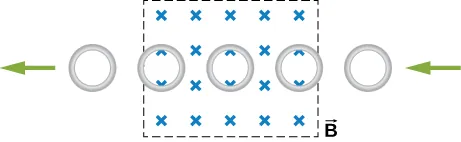14.

Show that $εε$ and $dΦm/dtdΦm/dt$ have the same units.

15.

State the direction of the induced current for each case shown below, observing from the side of the magnet.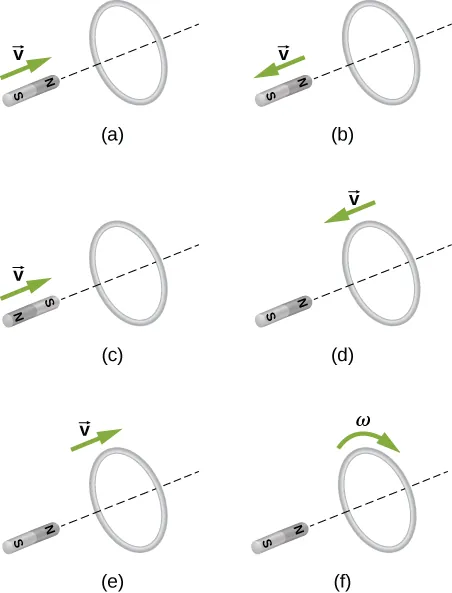### 13.3Motional Emf

16.

A bar magnet falls under the influence of gravity along the axis of a long copper tube. If air resistance is negligible, will there be a force to oppose the descent of the magnet? If so, will the magnet reach a terminal velocity?

17.

Around the geographic North Pole (or magnetic South Pole), Earth’s magnetic field is almost vertical. If an airplane is flying northward in this region, which side of the wing is positively charged and which is negatively charged?

18.

A wire loop moves translationally (no rotation) in a uniform magnetic field. Is there an emf induced in the loop?

### 13.4Induced Electric Fields

19.

Is the work required to accelerate a rod from rest to a speed v in a magnetic field greater than the final kinetic energy of the rod? Why?

20.

The copper sheet shown below is partially in a magnetic field. When it is pulled to the right, a resisting force pulls it to the left. Explain. What happen if the sheet is pushed to the left?### 13.5Eddy Currents

21.

A conducting sheet lies in a plane perpendicular to a magnetic field $B→B→$ that is below the sheet. If $B→B→$ oscillates at a high frequency and the conductor is made of a material of low resistivity, the region above the sheet is effectively shielded from $B→B→$. Explain why. Will the conductor shield this region from static magnetic fields?

22.

Electromagnetic braking can be achieved by applying a strong magnetic field to a spinning metal disk attached to a shaft. (a) How can a magnetic field slow the spinning of a disk? (b) Would the brakes work if the disk was made of plastic instead of metal?

23.

A coil is moved through a magnetic field as shown below. The field is uniform inside the rectangle and zero outside. What is the direction of the induced current and what is the direction of the magnetic force on the coil at each position shown?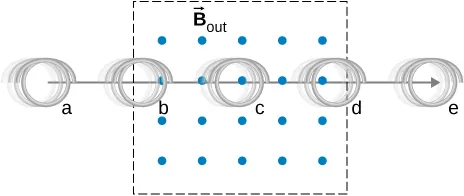Order a print copy

As an Amazon Associate we earn from qualifying purchases.